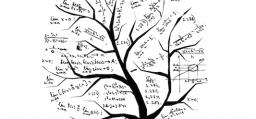# Amount 3791

X + Y = 31835
X is the amount stolen by 1,2 and 3
Y is the amount stolen by 4 and 5
1. stole half of X,
2. half the rest and 3 more on top of that
3. stole 6801.5
4. He stole 1/9 of Y, which is 513
5. He stole the rest

X =  27206
Y =  4629
a =  13603
b =  6801.5
c =  6801.5
d =  513
f =  4116

### Step-by-step explanation:

X + Y = 31835
X = a+b+c
Y = d + f
a=X/2
b = (X-a)/2
c = 6801.5
d = 513

X+Y = 31835
X-a-b-c = 0
Y-d-f = 0
X-2a = 0
X-a-2b = 0
c = 6801.5
d = 513

Row 2 - Row 1 → Row 2
X+Y = 31835
-Y-a-b-c = -31835
Y-d-f = 0
X-2a = 0
X-a-2b = 0
c = 6801.5
d = 513

Row 4 - Row 1 → Row 4
X+Y = 31835
-Y-a-b-c = -31835
Y-d-f = 0
-Y-2a = -31835
X-a-2b = 0
c = 6801.5
d = 513

Row 5 - Row 1 → Row 5
X+Y = 31835
-Y-a-b-c = -31835
Y-d-f = 0
-Y-2a = -31835
-Y-a-2b = -31835
c = 6801.5
d = 513

Row 3 + Row 2 → Row 3
X+Y = 31835
-Y-a-b-c = -31835
-a-b-c-d-f = -31835
-Y-2a = -31835
-Y-a-2b = -31835
c = 6801.5
d = 513

Row 4 - Row 2 → Row 4
X+Y = 31835
-Y-a-b-c = -31835
-a-b-c-d-f = -31835
-a+b+c = 0
-Y-a-2b = -31835
c = 6801.5
d = 513

Row 5 - Row 2 → Row 5
X+Y = 31835
-Y-a-b-c = -31835
-a-b-c-d-f = -31835
-a+b+c = 0
-b+c = 0
c = 6801.5
d = 513

Row 4 - Row 3 → Row 4
X+Y = 31835
-Y-a-b-c = -31835
-a-b-c-d-f = -31835
2b+2c+d+f = 31835
-b+c = 0
c = 6801.5
d = 513

Row 5 - -1/2 · Row 4 → Row 5
X+Y = 31835
-Y-a-b-c = -31835
-a-b-c-d-f = -31835
2b+2c+d+f = 31835
2c+0.5d+0.5f = 15917.5
c = 6801.5
d = 513

Row 6 - 1/2 · Row 5 → Row 6
X+Y = 31835
-Y-a-b-c = -31835
-a-b-c-d-f = -31835
2b+2c+d+f = 31835
2c+0.5d+0.5f = 15917.5
-0.25d-0.25f = -1157.25
d = 513

Pivot: Row 6 ↔ Row 7
X+Y = 31835
-Y-a-b-c = -31835
-a-b-c-d-f = -31835
2b+2c+d+f = 31835
2c+0.5d+0.5f = 15917.5
d = 513
-0.25d-0.25f = -1157.25

Row 7 - -0.25 · Row 6 → Row 7
X+Y = 31835
-Y-a-b-c = -31835
-a-b-c-d-f = -31835
2b+2c+d+f = 31835
2c+0.5d+0.5f = 15917.5
d = 513
-0.25f = -1029

f = -1029/-0.25 = 4116
d = 513/1 = 513
c = 15917.5-0.5d-0.5f/2 = 15917.5-0.5 · 513-0.5 · 4116/2 = 6801.5
b = 31835-2c-d-f/2 = 31835-2 · 6801.5-513-4116/2 = 6801.5
a = -31835+b+c+d+f/-1 = -31835+6801.5+6801.5+513+4116/-1 = 13603
Y = -31835+a+b+c/-1 = -31835+13603+6801.5+6801.5/-1 = 4629
X = 31835-Y = 31835-4629 = 27206

X = 27206
Y = 4629
a = 13603
b = 13603/2 = 6801.5
c = 13603/2 = 6801.5
d = 513
f = 4116

Our linear equations calculator calculates it.Did you find an error or inaccuracy? Feel free to write us. Thank you!

Tips for related online calculators
Do you have a linear equation or system of equations and looking for its solution? Or do you have a quadratic equation?